### 1. Preloaded examples in spin2

Chebfun's spin2 and spinop2 codes, like spin and spinop in 1D, include a number of preloaded examples, which you can find out about by typing help spin2 or help spinop2. One of the options is spin2('gl') for the 2D Complex Ginzburg-Landau equation. What is this equation?

To find out, you can type

S = spinop2('gl')
S =
spinop2 with properties:

domain: [0 100 0 100]
init: [InfxInf chebfun2]
lin: @(u)lap(u)
nonlin: @(u)u-(1+1.5i)*u.*(abs(u).^2)
tspan: [0 100]
numVars: 1


From here we see that the version of the equation in Chebfun is $$u_t = \Delta u + u - (1+1.5i)u |u|^2 .$$ Thus we have a scalar PDE involving a complex variable. This equation has been used by a number of authors in the study of chaotic processes arising in fluid mechanics and other areas [1,2].

Using the above as a model, let us set up our own complex Ginzburg-Landau calculation as follows.

dom = 50*[-1 1 -1 1];
tspan = [0 16];
S = spinop2(dom,tspan);
S.lin = @(u) lap(u);
S.nonlin = @(u) u - (1+1.5i)*u.*(abs(u).^2);

### 2. Non-chaotic solutions

For our first initial condition, suppose we take the complex function $$u_0(x,y) = (ix+y) \exp(-0.03(x^2+y^2)).$$ We let 16 time units time elapse and find a pretty spiral:

x = chebfun2(@(x,y) x,dom); y = chebfun2(@(x,y) y,dom);
u1 = (1i*x+y).*exp(-.03*(x.^2+y.^2)); S.init = u1;
npts = 80; dt = 4/npts; tic
u = spin2(S,npts,dt,'plot','off');
plot(real(u)), view(0,90), axis equal, axis offHere is the analogous experiment with the real initial condition $$u_0(x,y) = (x+y) \exp(-0.03(x^2+y^2)).$$

u2 = (x+y).*exp(-.03*(x.^2+y.^2)); S.init = u2;
u = spin2(S,npts,dt,'plot','off');
plot(real(u)), view(0,90), axis equal, axis offTime for the first pair of computations:

time_in_seconds = toc
time_in_seconds =
3.101127954000000


### 3. Beginnings of chaos

Spin computations always live on periodic domains. We now extend these two computations to $t=48$, time enough for the function values to pass across the boundary and come in the other side. Here is the first computation.

tspan = [0 48];
S = spinop2(dom,tspan);
S.lin = @(u) lap(u);
S.nonlin = @(u) u - (1+1.5i)*u.*(abs(u).^2);
S.init = u1; tic
u = spin2(S,npts,dt,'plot','off');
plot(real(u)), view(0,90), axis equal, axis off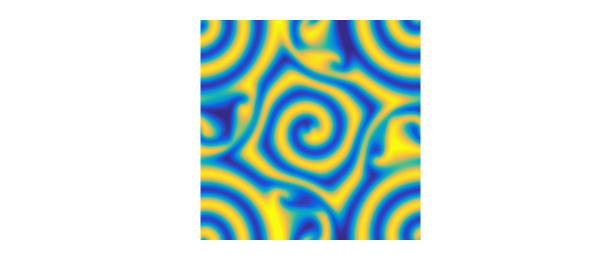The picture reveals the remnants of the spiral in the middle but some more complicated behavior in the corners. Experiments on a finer mesh show that this picture is correct. The structures have somewhat of a random appearance, but they are not random.

Now we run the second function to $t=48$. This image is also correct. Note the preservation of the diagonal line of symmetry.

S.init = u2;
u = spin2(S,npts,dt,'plot','off');
plot(real(u)), view(0,90), axis equal, axis off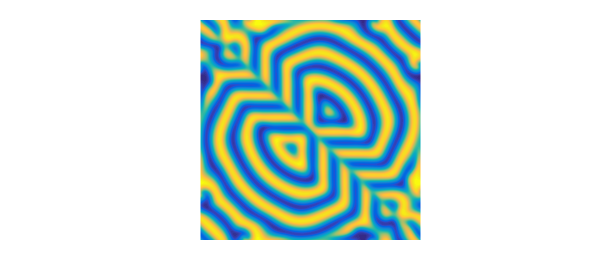Time for this second round of computations:

time_in_seconds = toc
time_in_seconds =
6.744747920000000


### 4. Chaos

Let's go now to $t=96$. Experiments with different grids confirm that this first image is correct.

tic, tspan = [0 96];
S = spinop2(dom,tspan);
S.lin = @(u) lap(u);
S.nonlin = @(u) u - (1+1.5i)*u.*(abs(u).^2);
S.init = u1;
tic, u = spin2(S,npts,dt,'plot','off');
plot(real(u)), view(0,90), axis equal, axis off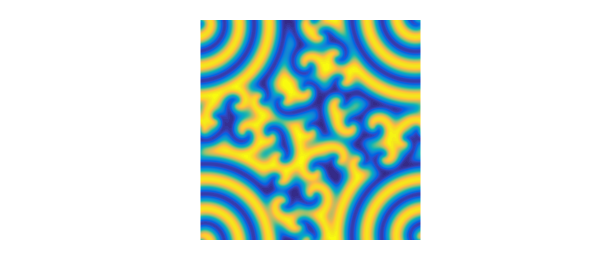With the second function, we refine the grid enough to get a plausible picture, but it is not in fact converged. So this image is perhaps physically correct, but not mathematically correct. Note that the symmetry line has been lost.

npts = 128; dt = 4/npts; tic
S.init = u2;
u = spin2(S,npts,dt,'plot','off');
plot(real(u)), view(0,90), axis equal, axis off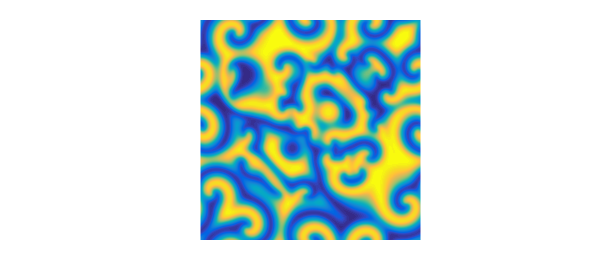Time for the third round of computations:

time_in_seconds = toc
time_in_seconds =
47.730490695999997


### 5. A bigger canvas

Let's double the size of the domain and the number of grid points in each direction. Here's the solution at $t=30$.

dom = 100*[-1 1 -1 1];
tspan = [0 30 60];
S = spinop2(dom,tspan);
S.lin = @(u) lap(u);
S.nonlin = @(u) u - (1+1.5i)*u.*(abs(u).^2);
x = chebfun2(@(x,y) x,dom); y = chebfun2(@(x,y) y,dom);
u1 = (1i*(x-8)+(y-2)).*exp(-.03*((x-8).^2+(y-2).^2)) + ...
((x+8)-(y+2)).*exp(-.03*((x+8).^2+(y+2).^2));
S.init = u1;
npts = 128; dt = 8/npts; tic
u = spin2(S,npts,dt,'plot','off');
plot(real(u{2})), view(0,90), axis equal, axis offAnd at $t=60$, of questionable accuracy:

plot(real(u{3})), view(0,90), axis equal, axis off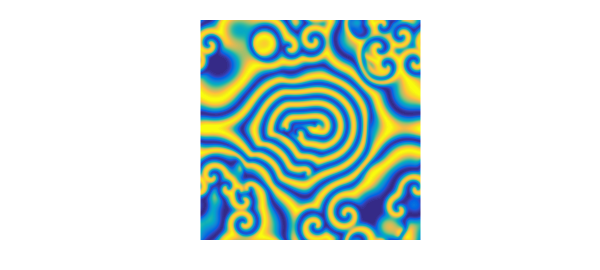To close a psychedelic note here is a phase portrait, obtained by plotting $u$ rather than its real part.

plot(u{3})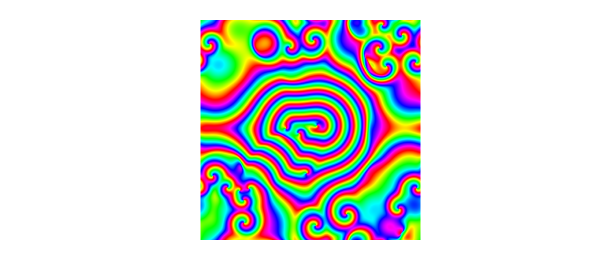Time for the big canvas computation:

time_in_seconds = toc
time_in_seconds =
19.036402233000000


### 6. References

 H. Montanelli and N. Bootland, Solving periodic semilinear stiff PDEs in 1D, 2D and 3D with exponential integrators, submitted, 2016.

 L. N. Trefethen and K. Embree, editors, The (Unfinished) PDE Coffee Table Book, https://people.maths.ox.ac.uk/trefethen/pdectb.html.